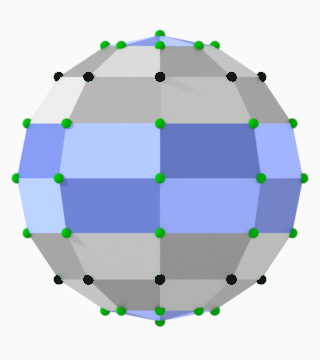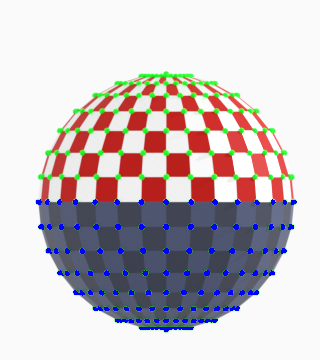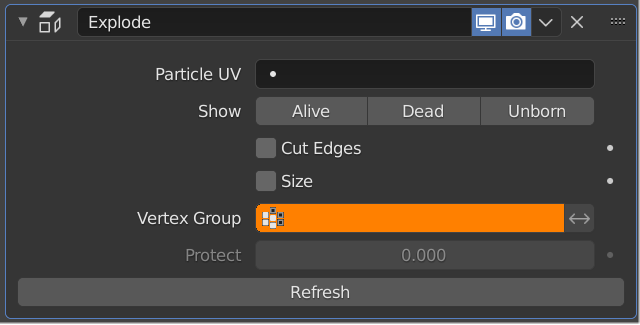# Vertex Group

– Vertices with full weight are not affected at all
– Vertices with less weight have a higher chance of being affected.
– Vertices with null weight will be treated like those which do not belong to the group at all, and explode normally.

– In this example only the blue faces (where the four vertexes weights are set to 0) can explode when the Vertex Group is selected

– Here you can see the Vertex Weights:
– Black vertexes: weight=1 (connected faces are sticked to these vertexes)
– Green vertexes: weight=0 (connected faces are free to ‘explode’)– Another example, changing the wertex weight of all vertexes connected to a grey face:

– Here you can see the Vertex Weights:
– Green vertexes: weight=0 (note how the upper faces, but some connected to blue vertexes, are free to ‘explode’)
– Blue vertexes: weight=0.1 (left video) and 0.4 (right video)